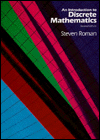##An Introduction to Discrete Mathematics

Designed for a freshman-sophomore course in Discrete Mathematics, with two goals in mind: To acquaint students with a variety of mathematical concepts that will be needed in the study of computer science; and to introduce students to the "mathematical" way of thinking, that is, the ideas of a definition, theorem and a proof. The book does not try to be encyclopedic in coverage.

### Contents

• Sets, Functions and Proof Techniques
• The Language of Sets
• One-to-one Correspondences
• Countable and Uncountable Sets (Optional)
• Functions
• Inductive Proofs and Inductive Definitions
• Logic and Logic Circuits
• Statements, Connectives, and Symbolic Language
• Truth Tables, Tautologies, and Contradictions
• Logical Equivalence
• Valid Arguments
• Boolean Functions and Disjunctive Normal Form
• Logic Circuits
• Karnaugh Maps
• Relations on Sets
• Relations
• Properties of Relations
• Equivalence Relations
• Partially Ordered Sets
• More on Partially Ordered Sets; Maximal and Minimal Elements and Topological Sorting
• Order Isomorphisms
• Combinatorics - The Art of Counting
• Introduction
• The Multiplication Rule
• The Pigeonhole Principle
• Permutations
• More on Permutations
• Combinations
• Properties of Binomial Coefficients
• The Multinomial Coefficient
• An Introduction to Recurrence Relations
• Second Order Linear Homogeneous Recurrence Relations with Constant Coefficients
• Second Order Linear Nonhomogeneous Recurrence Relations with Constant Coefficients
• Generating Functions and Recurrence Relations
• More on Combinatorics
• Permutations with Repetitions
• Combinations with Repetitions
• Linear Equations with Unit Coefficients
• Distributing Balls into Boxes
• The Principle of Inclusion-Exclusion I
• The Principle of Inclusion-Exclusion II
• The Principle of Inclusion-Exclusion III
• An Introduction to Probability
• An Introduction to Graph Theory
• Introduction
• Paths and Connectedness
• Eulerian and Hamiltonian Graphs
• Graph Isomorphisms; Planar Graphs
• Trees; The Depth First Search
• Two Applications of Trees: Binary Search Trees and Huffman Codes
• Undirected Networks; The Minimal Spanning Tree Problem
• Directed Graphs; Strong Connectivity
• Directed Networks: The Shortest Path Problem
• Finite State Machines

Second edition, 1989, ISBN 1-878015-29-X, 469 pp., Hardcover